### Home > CALC > Chapter 1 > Lesson 1.3.2 > Problem1-116

1-116.
1. Calculus problems often require using one or more of the trigonometric identities to solve problems. Solve each of the following equations where x ∈ [0, 2π]. Use exact values. Homework Help ✎

1. tan x · csc x = 2

2.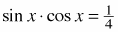3. 2 sin2 x − cos x − 1 = 0

4. tan x + cot x = −2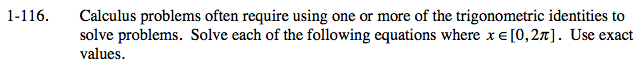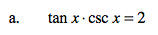Simplify the left side of the equation to solve for x.

$\text{Remember that tan}x=\frac{\text{sin}x}{\text{cos}x} \text{ and }\text{csc}x=\frac{1}{\text{sin}x}.$

$\frac{\text{sin}x}{\text{cos}x}\cdot\frac{1}{\text{sin}x}=2$

$\frac{1}{\text{cos}x}=2$

$\text{cos}x=\frac{1}{2}$

Use the unit circle to solve for x.

$\frac{\pi }{3},\ \frac{5\pi }{3}$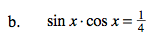See part (a).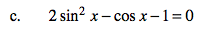See part (a).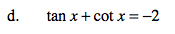See part (a).